Courses

# Magnetically Coupled Circuits - 1

## 10 Questions MCQ Test Topicwise Question Bank for Electronics Engineering | Magnetically Coupled Circuits - 1

Description
This mock test of Magnetically Coupled Circuits - 1 for Electrical Engineering (EE) helps you for every Electrical Engineering (EE) entrance exam. This contains 10 Multiple Choice Questions for Electrical Engineering (EE) Magnetically Coupled Circuits - 1 (mcq) to study with solutions a complete question bank. The solved questions answers in this Magnetically Coupled Circuits - 1 quiz give you a good mix of easy questions and tough questions. Electrical Engineering (EE) students definitely take this Magnetically Coupled Circuits - 1 exercise for a better result in the exam. You can find other Magnetically Coupled Circuits - 1 extra questions, long questions & short questions for Electrical Engineering (EE) on EduRev as well by searching above.
QUESTION: 1

Solution:
QUESTION: 2

### Two inductors of values L1 and L2 are coupled by a mutual inductance M. By interconnection of the two elements, one can have a minimum inductance of

Solution: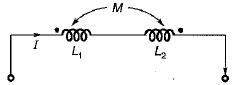When the effect of mutual inductance is subtractive then, we get minimum value of equivalent inductance, Leq = (L1 + L2 - 2M).

QUESTION: 3

### When two coupled coils of equal self inductance are connected in series in one way, the net inductance is 12 mH and when they are connected in the other way, the net inductance is 4 mH. The maximum value of net inductance when they are connected in parallel In a suitable way is

Solution:

Given,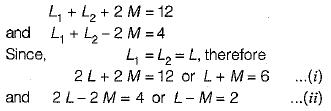From above two equations,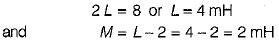To get maximum value in parallel connections,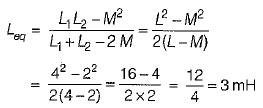QUESTION: 4

For the ideal transformer shown below, which of the following options is true?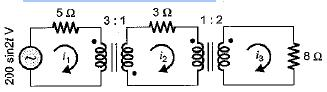Solution:

8 Ω resistance referred to middle circuit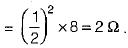Hence, total resistance in the middle circuit
=3 + 2= 5 Ω
This 5 Ω resistance referred to the first circuit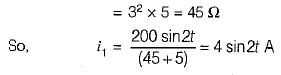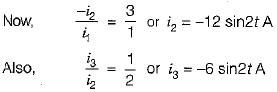QUESTION: 5

What the greatest mutual inductance that an air- core transformer can have with the selfinductances of 0.3 and 0.7 H respectively?

Solution: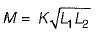For M to be maximum,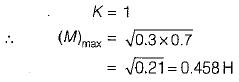QUESTION: 6

An air-core transformer has primary and secondary currents of i1= 0.2 A and i2 = 0.4 A that produces a flux of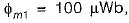,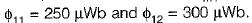. The turns ratio is N1: N2 = 25 : 40. What are the values of L1 and L2 in mH ?

Solution: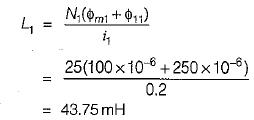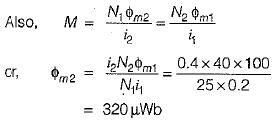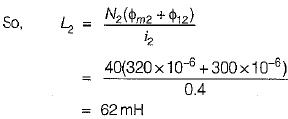QUESTION: 7

In an ideal transformer with an open-circuited secondary has inductances of L1 = 20 mH, L2 = 32 mH and M = 13 mH. The values of primary and secondary voltages when the primary current is increased at the rate of 0.4 kA/s will be respectively

Solution: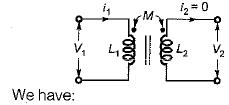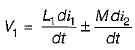(Here, polarity of M is not given in question Hence, it can be +ve or -ve)

andSince secondary is open-circuited, therefore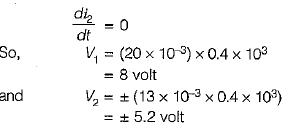QUESTION: 8

The input inductance of the circuit for ω = 1 rad/s is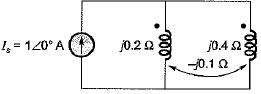Solution:

Let the voltage drop across the windings be V volt as shown below.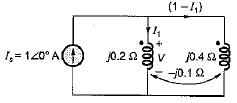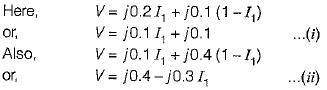(In both the equations above, the effect of mutual inductance is positive since current enters both the dots simultaneously).
On solving equation (i) and (ii), we get

V = j0.175 volt
Let the input inductance be L then,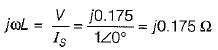∴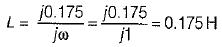QUESTION: 9

In a certain magnetic circuit, a current of 1 A produces a flux of 1 Wb. if the linear dimensions of the magnetic circuit are doubled, then for producing the same flux, the current would be

Solution:

We know that,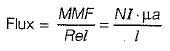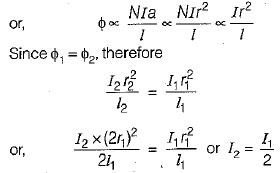Hence , the current will be come half i.e I2 = 1/2 A

QUESTION: 10

Mutual inductance between two magnetically coupled coils depends on the

Solution: